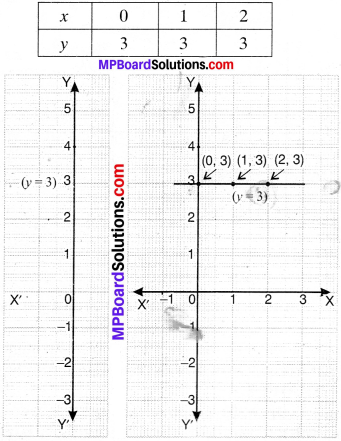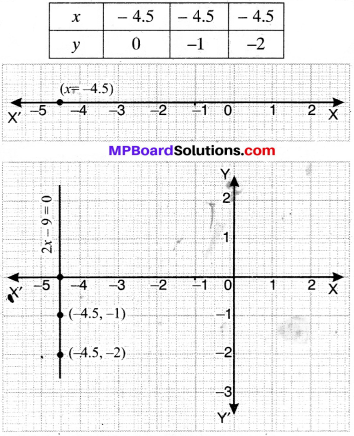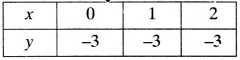## MP Board Class 9th Maths Solutions Chapter 4 Linear Equations in Two Variables Ex 4.4

Question 1.
Give the geometric representations of y = 3 as an equation –

1. In one variable
2. in two variables.

Solution:
1. Linear equation in one variable
y = 3

2. Linear equation in two variables is
0x + y – 3 = 6Question 2.
Give the geometric representation of 2x + 9 = 0 as an equation –

1. In one variable
2. In two variables.

Solution:
1. Linear equation in one variable
2x + 9 = 0
2x = – 9
x = – 4.5

2. Linear equation in two variables
2x + 0y + 9 = 0Equations of a Line Parallel to the x – axis and y – axis:
This is a special case when the given point lies on the axes, either x – axis or y – axis. If the point lies on x – axis, then y – coordinate will be 0 and if the point lies on y-axis, then the x-coordinate will be 0.Example 1.
Draw the graph of the equation represented by a straight line which is parallel to the x – axis and at a distance 3 units below it. (NCERT Exemplar)
Solution:
The equation of a line which is parallel to the x-axis and at a distance of 3 units below. It is given by
y = – 3
The solutions of the equation are:The graph is shown below.